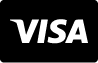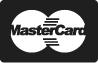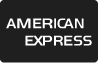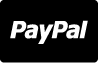# 免版税图像 - Equations background with geometry, physics, maths and trigonometry scientific formulas. Vector green chalkboard with data lettering, algorithms and logarithms, integrals and theorems, cos and sin

Equations background with geometry, physics, maths and trigonometry scientific formulas. Vector green chalkboard with data lettering, algorithms and logarithms, integrals and theorems, cos and sin

5000 x 3750 px | 16.67 " x 12.5 " | 300dpi | JPG

1 866 655 3733